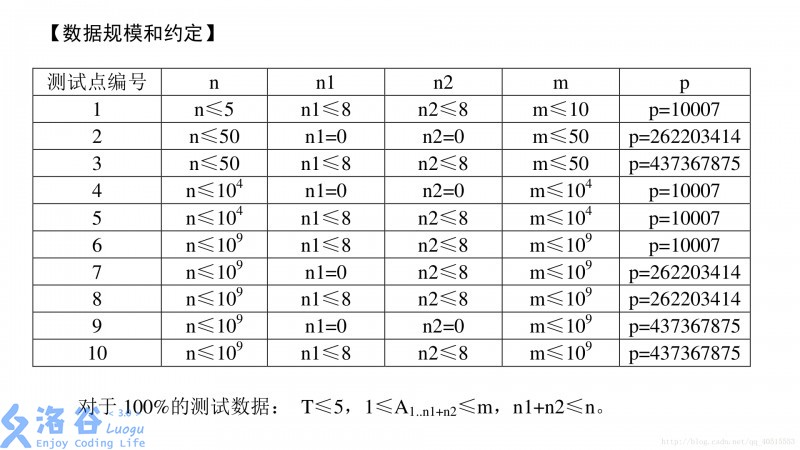# P3301 [SDOI2013]方程

• 152通过
• 770提交
• 题目提供者 洛谷
• 评测方式 云端评测
• 标签 同余,中国剩余定理 容斥 组合数学 2013 山东 高性能
• 难度 省选/NOI-
• 时空限制 1000ms-2000ms / 128MB
• 提示：收藏到任务计划后，可在首页查看。

## 题目描述

给定方程

$$X_1+X_2+. +X_n=M$$ 我们对第l..N1个变量进行一些限制：

$X_{1} \le A_{1}$
$X_{2} \le A_{2}$
$...$
$X_{n1} \le A_{n1}$

我们对第n1 + 1..n1+n2个变量进行一些限制：

$X_{n1+l} \ge A_{n1+1}$
$X_{n1+2} \ge A_{n1+2}$
$...$
$X_{n1+n2} \ge A_{n1+n2}$

求：在满足这些限制的前提下，该方程正整数解的个数。答案可能很大，请输出对p取模后的答案，也即答案除以p的余数。

## 输入输出格式

输入格式：

输入含有多组数据。

第一行两个正整数T，p。T表示这个测试点内的数据组数，p的含义见题目描述。

对于每组数据，第一行四个非负整数n，n1，n2，m。
第二行nl+n2个正整数，表示A1..n1+n2。请注意，如果n1+n2等于0，那么这一行会成为一个空行。

输出格式：

共T行，每行一个正整数表示取模后的答案。

## 输入输出样例

输入样例#1： 复制
3 10007
3 1 1 6
3 3
3 0 0 5

3 1 1 3
3 3
输出样例#1： 复制
3
6
0



## 说明

【样例说明】 对于第一组数据，三组解为(1，3，2)，(1,4,1),(2,3,1) 对于第二组数据，六组解为(1，1，3)，(1,2,2),(1,3,1),(2,1,2),(2,2,1),(3,1,1)n < = 10^9 , n1 < = 8 , n2 < = 8 , m < = 10^9 ,p<=437367875

对于l00%的测试数据： T < = 5，1 < = A1..n1_n2 < = m，n1+n2 < = n

提示
标程仅供做题后或实在无思路时参考。
请自觉、自律地使用该功能并请对自己的学习负责。
如果发现恶意抄袭标程，将按照I类违反进行处理。